# Chapter 4 : HYDROLOGY¶

## Example 4.1 pg : 113¶

In :

#Given
p = [78.8, 90.2, 98.6, 102.4, 70.4];   				#rain guage readings at respective stations
s = 0.;

# Calculations and Results
for i in range(5):
s = s+p[i];

pavg = s/5;
u = 0;
for i in range(5):
u = u+(p[i]-pavg)**2;

sx = (u/4)**0.5;
Cv = sx*100/pavg;
N = (Cv/6)**2;
N = round(N*100)/100;
print "mean rainfall = %.2f cm."%(pavg);
print "total stations needed = %.2f."%(N);
#taking N = 7
N = 7;
n = N-5;
print "additional guages needed = %i."%(n);

mean rainfall = 88.08 cm.
total stations needed = 6.44.
additional guages needed = 2.


## Example 4.2 pg : 115¶

In :

#Given
pB = 48.;         				#precipitation at B
pC = 51.;         				#precpitation at C
pD = 45.;         				#precipitation at D

# Calculations
pA = (pB+pC+pD)/3;

# Results
print "precipitation at A = %i mm."%(pA);

precipitation at A = 48 mm.


## Example 4.3 pg : 115¶

In :

#Given
pA = 6.6;         				#precipitation at A
pB = 4.8;         				#precpitation at B
pC = 3.7;         				#precipitation at C
nA = 72.6;        				#normal precipitation at A
nB = 51.8;        				#normal precipitation at B
nC = 38.2;        				#normal precipitation at C
nX = 65.6;        				#normal precipitation at X

# Calculations
pX = (nX*pA/nA+nX*pB/nB+nX*pC/nC)/3;
pX = round(pX*100)/100;

# Results
print "precipitation at x = %.2f cm."%(pX);

precipitation at x = 6.13 cm.


## Example 4.4 pg : 115¶

In :

#Given
pB = 74.;         				#precipitation at B
pC = 88.;         				#precpitation at C
pD = 71.;         				#precipitation at D
pE = 80.;         				#precipitation at E
Bx = 9.;
By = 6.;
Cx = 12.;
Cy = -9.;
Dx = -11.;
Dy = -6.;
Ex = -7.;
Ey = 7.;
Ax = 0;
Ay = 0;

# Calculations
Db = (Bx**2+By**2);
Dc = (Cx**2+Cy**2);
Dd = (Dx**2+Dy**2);
De = (Ex**2+Ey**2);
Wb = 1/Db;
Wc = 1/Dc;
Wd = 1/Dd;
We = 1/De;
s = pB*Wb+pC*Wc+pD*Wd+pE*We;
pA = s/(Wb+Wc+Wd+We);
pA = round(pA*10)/10;

# Results
print "precipitation at A = %.2f mm."%(pA);

precipitation at A = 77.50 mm.


## Example 4.5 pg : 119¶

In :
import math
from numpy import zeros

#Given
p = [58., 61., 69., 56., 84., 86., 69., 79., 71.];   				#values of precipitation
s = 0;

# Calculations and Results
for i in range(9):
s = s+p[i];
ar = s/9;
ar = round(ar*10)/10;
print "umath.sing arithmatic average method:"
print "Average rainfall = %.2f cm."%(ar);

I = [86., 85., 80., 75., 70., 65., 60., 55., 50.];          				#isphytes
A = [0.43, 5.20, 4.0, 5.04, 5.85, 4.53, 4.09, 1.27];  				#area between isohytes

a = zeros(9)
for i in range(8):
a[i] = (I[i]+I[i+1])/2;

P = zeros(8)
for i in range(8):
P[i] = A[i]*a[i];

s = 0;
for i in range(8):
s = s+P[i];

t = 0;
for i in range(8):
t = t+A[i];

ar = s/t;
ar = round(ar*10)/10;
print "isohytel method:"
print "Average rainfall = %.2f cm."%(ar);

A = [3.26, 0.39, 1.61, 2.04, 2.46, 0.84, 3.91, 5.09, 0.41, 3.94, 2.06, 4.40];   				#thiessen area
p = [58., 63., 71., 69., 86., 81., 84., 56., 53., 69., 61., 79.];           				#observed precipitation
P = zeros(12)
for i in range(12):
P[i] = A[i]*p[i];

s = 0;
for i in range(12):
s = s+P[i];

t = 0;
for i in range(12):
t = t+A[i];

ar = s/t;
ar = round(ar*10)/10;
print "thiesson polygon method:"
print "Average rainfall = %.2f cm."%(ar);

#mean rainfall obtained by thiesson polygon method is different from book as product(A*P) is round offed in book.

umath.sing arithmatic average method:
Average rainfall = 70.30 cm.
isohytel method:
Average rainfall = 69.70 cm.
thiesson polygon method:
Average rainfall = 70.00 cm.


## Example 4.6 pg : 127¶

In :
%matplotlib inline
import math
from matplotlib.pyplot import plot
from numpy import zeros

#Given
X = [69., 55., 62., 67., 87., 70., 65., 75., 90., 100., 90., 95., 85., 90., 75., 95.];   				#annual rainfall at X
Y = [77., 62., 67., 68., 86., 90., 65., 75, 70, 70, 70 ,75 ,65 ,70, 55, 75];    				#average rainfall at 10 base stations
cx = zeros(16)
cx = 69;               				#accumulated annual values at station X
for i in range(1,16):
cx[i] = cx[i-1]+X[i];

cy = zeros(16)
cy = 77;
for i in range(1,16):
cy[i] = cy[i-1]+Y[i];  				#accumulated annual values at ten stations

#since curve is not having unform slope
print "Record at X is not consistent.";
print "From the curve regime is observed in the year 1978."

Q = [1970., 1971., 1972., 1973., 1974., 1975., 1976., 1977.];
O = [95., 75., 90., 85., 95., 90., 100., 90.];
A = zeros(8)
for i in range(8):
A[i] = 0.7051*O[i];

print "Year          Observed rainfall          Adjusted rainfall";
for i in range(8):
print "%i                %i                     %i"%(Q[i],O[i],A[i]);

#graph is plotted between cx and cy
plot(cy,cx,cy,cx,"ro")

Record at X is not consistent.
From the curve regime is observed in the year 1978.
Year          Observed rainfall          Adjusted rainfall
1970                95                     66
1971                75                     52
1972                90                     63
1973                85                     59
1974                95                     66
1975                90                     63
1976                100                     70
1977                90                     63

Out:
[<matplotlib.lines.Line2D at 0x107357550>,
<matplotlib.lines.Line2D at 0x1073577d0>]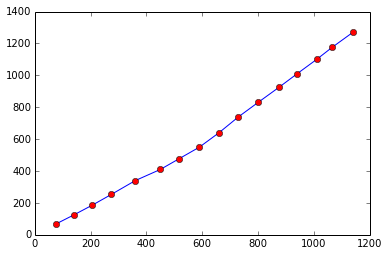## Example 4.7 pg : 130¶

In :
%matplotlib inline
from matplotlib.pylab import bar,xlabel,ylabel
from numpy import zeros,linspace

#Given
c = [0, 12.4, 22.1, 35.1, 52.7, 63.7, 81.9, 109.2, 123.5, 132.6, 143.3, 146., 146.];  				#cumulative rainfall
T = linspace(0,13,len(c))          				#Time
t = 15./60;            				#time interval
r = zeros(13)
I = zeros(13)
r = 0;
print "Rainfall intensity:";
I = 0;
for i in range(1,13):
r[i] = c[i]-c[i-1];
I[i] = r[i]/t;          				#Rainfall intensity
print "%.2f"%(I[i]);

#graph is plotted between I and T
bar(T,I)
xlabel("Time hr")
ylabel("Rain fall insentity")

Rainfall intensity:
49.60
38.80
52.00
70.40
44.00
72.80
109.20
57.20
36.40
42.80
10.80
0.00

Out:
<matplotlib.text.Text at 0x1073839d0>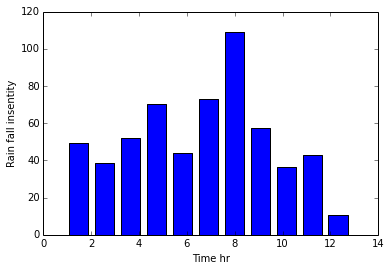## Example 4.8 pg : 131¶

In :
%matplotlib inline
import math
from numpy import zeros_like,array
from matplotlib.pylab import plot,xlabel,ylabel

#Given
CR = array([0, 12.4, 22.1 ,35.1, 52.7, 63.7, 81.9, 109.2, 123.5, 132.6, 143.3, 146.0, 146.0]);  				#cumulative rainfall

c15 = zeros_like(CR)
c30 = zeros_like(CR)
c45 = zeros_like(CR)
c60 = zeros_like(CR)
c90 = zeros_like(CR)
c120 = zeros_like(CR)

# Calculations and Results
c15 = 12.4;
c30 = 22.1;
c45 = 35.1;
c60 = 52.7;
c90 = 81.9;
c120 = 123.5;
for i in range(2,13):
c15[i] = CR[i]-CR[i-1];

for i in range(3,13):
c30[i] = CR[i]-CR[i-2];

for i in range(4,13):
c45[i] = CR[i]-CR[i-3];

for i in range(5,13):
c60[i] = CR[i]-CR[i-4];

for i in range(7,13):
c90[i] = CR[i]-CR[i-6];

for i in range(9,13):
c120[i] = CR[i]-CR[i-8];

print "15min               30min               45min              60min          90min          120min";
for i in range(13):
print "%.2f          %.2f          %.2f          %.2f          %.2f          %.2f"%(c15[i],c30[i],c45[i],c60[i],c90[i],c120[i]);

I = [109.2, 91, 79.7, 74.1, 67.6, 61.75];    				#maximum intensity at respective durations
D = [15 ,30 ,45 ,60 ,90 ,120];               				#durations
#greph is plotted between I and D
plot(D,I,D,I,"ro")
xlabel("Duration")
ylabel("max rain fall intensity")

15min               30min               45min              60min          90min          120min
0.00          0.00          0.00          0.00          0.00          0.00
12.40          0.00          0.00          0.00          0.00          0.00
9.70          22.10          0.00          0.00          0.00          0.00
13.00          22.70          35.10          0.00          0.00          0.00
17.60          30.60          40.30          52.70          0.00          0.00
11.00          28.60          41.60          51.30          0.00          0.00
18.20          29.20          46.80          59.80          81.90          0.00
27.30          45.50          56.50          74.10          96.80          0.00
14.30          41.60          59.80          70.80          101.40          123.50
9.10          23.40          50.70          68.90          97.50          120.20
10.70          19.80          34.10          61.40          90.60          121.20
2.70          13.40          22.50          36.80          82.30          110.90
0.00          2.70          13.40          22.50          64.10          93.30

Out:
<matplotlib.text.Text at 0x10741e950>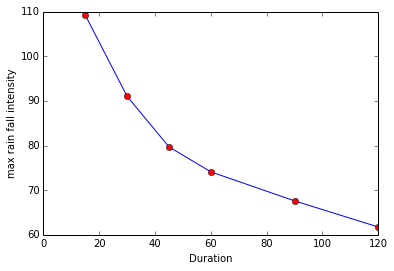## Example 4.9 pg : 132¶

In :
#Given
p = [475, 377, 731, 1066, 361, 305, 926, 628, 409, 236, 337, 853];   				#precipitation value
N = 12.;                       				#total number of years
T = 6;                        				#recurrence interval

# Calculations
m = N/T;

# Results
print "Ranking of storm = %i."%(m);
#hence pick 2nd severest storm
print "preciptation value which has recurrence period of 6 years = %i mm."%(p);

Ranking of storm = 2.
preciptation value which has recurrence period of 6 years = 926 mm.


## Example 4.10 pg : 132¶

In :
%matplotlib inline
import math
from numpy import arange,array,zeros_like

#Given
I = linspace(25,16,10)          				#isohytes
a = array([407 ,1008, 1522, 1909, 2216, 2460, 2651, 2782, 2910, 2936]);  				#enclosed area
ia = zeros_like(a)
ia = 407.;

# Calculations
for i in range(1,10):
ia[i] = a[i]-a[i-1];
r = linspace(25.5,16.5,10)
rv = r*ia

cv = zeros_like(rv)
cv = 10378;
for i in range(1,10):
cv[i] = cv[i-1]+rv[i];

eud = cv/a
print "From depth area curve we obtain average depth of precipitation = 24.1 mm for an area of 1800 sq. km.";
#graph is plotted between eud and a.
# Results
plot(a,eud,a,eud,"ro")
xlabel("Area")
ylabel("mean precipitation depth")

From depth area curve we obtain average depth of precipitation = 24.1 mm for an area of 1800 sq. km.

Out:
<matplotlib.text.Text at 0x1074d2250>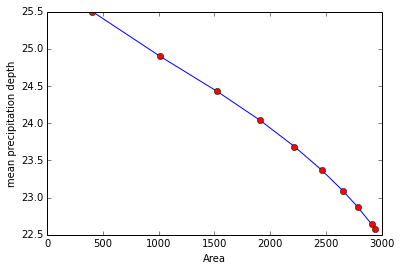## Example 4.11 pg :133¶

In :
%matplotlib inline
import math
from numpy import array,float64

#24h max. rainfall with return period of 8,15 and 25.
#24h max rainfall with 40%,24% and 8% probability.
#probabilty of rainfall of magnitude equal to or exceeding 100 mm.

#Given
N = 20.;
r = array([142, 126, 116, 108, 102, 95, 92, 88, 86, 82, 80, 78, 76, 73, 71, 69, 68, 66, 65, 64],dtype=float64);   				#rainfall in respective years
m = linspace(1,20,20)             				#ranking of storm
p = m*100/(N+1)
T = 100/p

# Calculations and Results
#from frequency curve obtained we get
#Part (a)
T1 = array([8, 15, 25]);
r1 = array([119, 134, 149]);
print "Tyears          Rainfallmm";
for i in range(3):
print "%i                         %i"%(T1[i],r1[i]);

#Part (b)
p1 = [40 ,24, 8];
r2 = [87, 101, 130];
print "probabilitypercent          Rainfallmm";
for i in range(3):
print "%i                                %i"%(p1[i],r2[i]);

print "For rainfall = 100 m.T = 4 years.Probability = 25 percent.";

Tyears          Rainfallmm
8                         119
15                         134
25                         149
probabilitypercent          Rainfallmm
40                                87
24                                101
8                                130
For rainfall = 100 m.T = 4 years.Probability = 25 percent.


## Example 4.12 pg : 135¶

In :
%matplotlib inline
import math
from matplotlib.pylab import plot,xlabel,ylabel
from numpy import array,float64

#plot IDF curve for return period of 10,2 and 1 years umath.sing california formula

#Given
t = array([5, 10, 20, 30, 60, 90, 120],dtype=float64);       				#duration
#value of P for respective return period is
p10 = array([10.6, 14.7, 19.3, 20.8, 25.5, 29, 34.7]);  				#rainfall for T = 10 years
p2 = array([8.2, 10.3, 13.2, 14.2, 16.6 ,19.4, 21.4]);  				#rainfall for T = 2 years
p1 = array([3.5, 6.2, 8.9, 10, 13.2, 15, 16.5]);        				#rainfall for T = 1 year

# Calculations
i1 = p10*60/t;                				#intensity of rainfall with return period of 10 years
i2 = p2*60/t;                 				#intensity of rainfall with return period of 2 years
i3 = p1*60/t;                 				#intensity of rainfall with return period of 1 year

# Results
#graph is plotted between #t and i1 #t and i2 #t and i3
plot(t,i1)
plot(t,i2)
plot(t,i3)
xlabel("Duration")
ylabel("Intensity")

Out:
<matplotlib.text.Text at 0x107511cd0>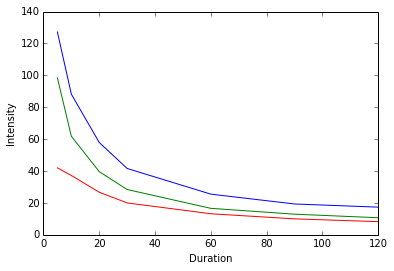## Example 4.13 pg : 137¶

In :
%matplotlib inline
import math
from numpy import linspace,array
from matplotlib.pylab import subplot,plot,xlabel,ylabel

#Given
N = 20.;
m = linspace(1,20,20)             				#rank number
rd = array([82, 78, 75, 72, 70, 68, 65, 63, 61, 58, 56, 54, 52, 50, 46, 40, 36, 34, 32, 30]);   				#rainfall in decremath.sing order

# Calculations
ri = rd[::-1]
T = N/(m-0.5);

# Results
#from the curves
print "maximum rainfall = 79cm for T = 15 years.";
print "minimum rainfall  = 31 cm for T = 15 years.";
#graph is plotted between rd and T;ri and T
subplot(121)
plot(T,rd)
xlabel("Reccurance interval")
ylabel("rainfall cm")
subplot(122)
plot(T,ri)
xlabel("Reccurance interval")
ylabel("rainfall cm")

maximum rainfall = 79cm for T = 15 years.
minimum rainfall  = 31 cm for T = 15 years.

Out:
<matplotlib.text.Text at 0x107643c10>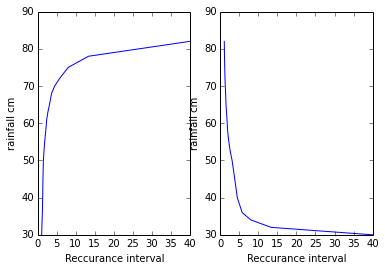## Example 4.14 pg : 144¶

In :
import math
from numpy import zeros
#average evaporation for one week

#Given
w = [12, 5, 2, -3, 1, 6, 11];     				#water added or taken out
r = [0, 6, 8, 12, 9, 5, 0];       				#rainfall
pan = zeros(7)
le = zeros(7)
for i in range(7):
pan[i] = w[i]+r[i];     				#Pan evaporation
le[i] = 0.8*pan[i];     				#lake evaporation
s = sum(le)

print "daily lake evaporationmm:";
for i in range(7):
print "%.2f"%(le[i]);

av = s/7;
av = round(av*100)/100;
print "average evaporation for one week = %.2f mm."%(av);

daily lake evaporationmm:
9.60
8.80
8.00
7.20
8.00
8.80
8.80
average evaporation for one week = 8.46 mm.


## Example 4.15 pg : 145¶

In :
import math

#total depth and volume of evaporation loss

#Given
Rh = 0.4;         				#relative humidity
A = 4.8;          				#average surface spread of reservior
v3 = 18.;          				#wind velocity at 3m above ground
es = 31.81;       				#saturated vapour pressure
Km = 0.36;        				#for large deep waters

# Calculations and Results
#umath.sing Meyer's formula
ea = es*Rh;
v9 = v3*(9./3)**(1./7);
E = Km*(es-ea)*(1+v9/16);
d = 7*E;
v = d*A*100/1000;
E = round(E*10)/10;
d = round(d*10)/10;
v = round(v*100)/100;
print "umath.sing Meyers formula:";
print "average evaporation loss from reservior = %.2f mm/day."%(E);
print "total depth = %.2f mm"%(d);
print "total volume = %.2f hectare-m."%(v);

#umath.sing Rohwer's formula
Pa = 760.;
vdash = (0.6/2)**(1./7)*18;
E = 0.771*(1.465-0.000732*Pa)*(0.44+0.0733*vdash)*(es-ea);
d = 7*E;
v = d*A*100/1000;
E = round(E*10)/10;
d = round(d*10)/10;
v = round(v*10)/10;
print "umath.sing Rohwers formula:";
print "average evaporation loss from reservior = %.2f mm/day."%(E);
print "total depth = %.2f mm"%(d);
print "total volume = %.2f hectare-m."%(v);

umath.sing Meyers formula:
average evaporation loss from reservior = 15.90 mm/day.
total depth = 111.40 mm
total volume = 53.47 hectare-m.
umath.sing Rohwers formula:
average evaporation loss from reservior = 20.70 mm/day.
total depth = 145.20 mm
total volume = 69.70 hectare-m.


## Example 4.16 pg : 149¶

In :
%matplotlib inline
import math
from numpy import array,zeros_like
from matplotlib.pylab import plot,xlabel,ylabel

#plot infiltration capacity curve

#Given
D = 30.;          				#diameter of inside ring of infiltrometer
A = math.pi*D**2/4;
V = array([0, 200, 470, 840, 1405, 1840, 2245, 2510, 2745, 2885, 2990, 3130, 3270],dtype=float64);  				#cumulative volume;
t = array([0, 2, 5, 10, 20, 30, 45, 60, 80, 100, 120, 150, 180],dtype=float64);                  				#Time(minutes)

# Calculations and Results
dt = zeros_like(t)

for i in range(1,13):
dt[i] = (t[i]-t[i-1])/60;

F = V/A;

Fd = zeros_like(F)
Fd = F;
for i in range(1,13):
Fd[i] = F[i]-F[i-1];

ft = Fd/dt     				#infirltration rate

#from the graph
print "constant rate of infiltration = 0.40 cm/hr.";
avg10 = F*60/10;
avg30 = F*60/30;
avg10 = round(avg10*100)/100;
avg30 = round(avg30*100)/100;
print "average rate of infiltration for first 10 min = %.2f cm/hr."%(avg10);
print "average rate of infiltration for first 30 min = %.2f cm/hr."%(avg30);
#graph is plotted between ft and t
plot(t,ft)
xlabel("time in mins")
ylabel("Infiltrtion rate")

constant rate of infiltration = 0.40 cm/hr.
average rate of infiltration for first 10 min = 7.13 cm/hr.
average rate of infiltration for first 30 min = 5.21 cm/hr.

Out:
<matplotlib.text.Text at 0x107365850>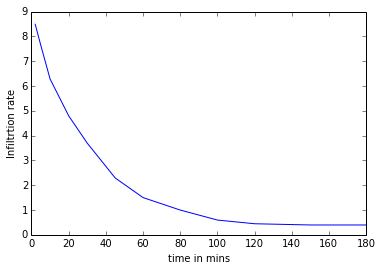## Example 4.17 pg : 162¶

In :
#drainage desity
#form factor
#channel slope
#average overland flow length

#Given
A = 82.;         				#area of watershed
d = 12.6;       				#dismath.tance between outlet and farther most point
l = 440.;        				#total length of channel
e = 656.;        				#elevation differnce between outlet and further most point

# Calculations
Dd = l/A;
ff = A/d**2;
cs = e/(d*1000);
lo = 1000/(2*Dd);
Dd = round(Dd*100)/100;
ff = round(ff*1000)/1000;

# Results
print "drainage desity = %.2f km/square.km."%(Dd);
print "form factor = %.2f."%(ff);
print "channel slope = %.2f."%(cs);
print "average overland flow length = %i m."%(lo);

drainage desity = 5.37 km/square.km.
form factor = 0.52.
channel slope = 0.05.
average overland flow length = 93 m.


## Example 4.18 pg : 163¶

In :
import math

#compute fi and W index

#Given
R = 3.6;        				#surface runoff
r = [0, 1.3, 2.8, 4.1, 3.9, 2.8, 2.0, 1.8, 0.9];  				#rainfall at respective time
t = 4.;             				#total time
s = sum(r[2:]);

# Calculations and Results
fi = (s-R*2)/6;
#math.since fi >1.3 and <1.8
print "fi index = %.2f cm."%(fi);
print "computations are correct.";

s = sum(r);
P = s/2;
Sr = 0.;
W = (P-R-Sr)/t;
print "W index = %.2f cm/hr."%(W);

fi index = 1.85 cm.
computations are correct.
W index = 1.55 cm/hr.


## Example 4.19 pg : 163¶

In :
import math
from numpy import linspace,array

#Given
T = linspace(1,9,9)      				#time from start
r = array([0.7, 1.4, 2.4, 3.7, 2.9, 2.6, 1.7, 0.8, 0.5]);  				#increamental rainfall
R = 9.3;        				#total run-off
s = sum(r)

ti = s-R;
#first trial
tr = 9.;   				#assumed
fi1 = ti/tr;
#this makes 1st,8th and 9th hour ineffective

#second trial
tr = 6.;
ti = s-R-r-r-r;
fi = ti/tr;
P = zeros_like(r)
for i in range(9):
P[i] = r[i]-fi;
if (P[i]<0):
P[i] = 0;

print "Timeh          rainfall excess.";
for i in range(9):
print "%.2f          %.2f"%(T[i],P[i]);

print "fi index = %.2f cm/hr."%(fi);
print "time of rainfall excess = %i hours.."%(tr);

Timeh          rainfall excess.
1.00          0.00
2.00          0.50
3.00          1.50
4.00          2.80
5.00          2.00
6.00          1.70
7.00          0.80
8.00          0.00
9.00          0.00
fi index = 0.90 cm/hr.
time of rainfall excess = 6 hours..


## Example 4.20 pg : 164¶

In :
from numpy import array
import math

#Given
P = array([72.2, 70.1, 73.3, 42.5, 81.3, 50.6, 52.9, 59.4, 60.3, 64.3, 68.8, 56.7, 77.2, 40.5, 44.1, 65.5]);  				#Precipitation
R = array([24.1, 22.7, 25.6, 11.3, 28.4, 12.7, 13.4, 15.7, 16.2, 17.7, 19.2, 14.9, 25.4, 10.6, 11.7, 17.9]);  				#runoff

# Calculations
Ps = P**2
Rs = R**2;
PR = P*R;

s = sum(Ps)
t = sum(Rs)
u = sum(PR)
q = sum(P)
w = sum(R)
N = 16.;
a = (N*u-q*w)/(N*s-q**2);
b = (w-a*q)/N;
b = round(b*1000)/1000;
a = round(a*10000)/10000;

# Results
print "Equation is:%.4f P %.3f."%(a,b);

Equation is:0.4375 P -8.823.


## Example 4.21 pg : 165¶

In :
%matplotlib inline
from numpy import linspace,array
import math
from matplotlib.pylab import plot,xlabel,ylabel

#Given
A = 8.6;                                    				#catchment area
T = linspace(0,4,9)                              				#time
r = array([0, 0.4, 1.1, 2.3, 3.8, 4.8, 5.6, 6.2, 6.7]);    				#accumulated rainfall
fi = 0.4;                                   				#fi index
dt = 0.5;                                   				#time interval

# Calculations and Results
d = zeros_like(r)

for i in range(1,9):
d[i] = r[i]-r[i-1];                 				#accumulated rainfall

print "Intensity of effective Rainfall:";
I = zeros_like(r)
p = zeros_like(r)
s = 0;
for i in range(1,9):
p[i] = d[i]-fi;                				#effective rainfall
I[i] = p[i]/dt;                				#Intensity of effective Rainfall
s = s+I[i];
print "%.2f"%(I[i]);

#graph is plotted between I and T
run = s*dt;
V = run*A*10000;
print "Volume of direct run-off = %.2f cubic metre."%(V);
plot(T,I)

Intensity of effective Rainfall:
0.00
0.60
1.60
2.20
1.20
0.80
0.40
0.20
Volume of direct run-off = 301000.00 cubic metre.

Out:
[<matplotlib.lines.Line2D at 0x1078dcf10>]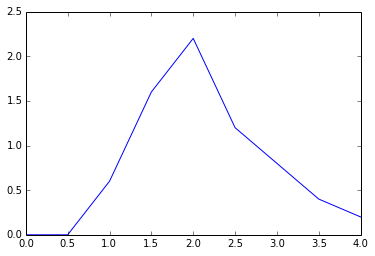## Example 4.22 pg : 166¶

In :
%matplotlib inline
from numpy import array,linspace
#total rainfall
#total rainfall excess
#W index

#Given
r = array([3.5, 6.5, 8.5 ,7.8, 6.4, 4, 4, 6]);       				#rainfall intensity
T = linspace(0,240,8)                        				#time
dt = 30.;                              				#time interval

# Calculations
s = sum(r);
P = s*dt/60;
Pe = ((6.5-4.5)+(8.5-4.5)+(7.8-4.5)+(6.4-4.5)+(6-4.5))*dt/60;    				#area of graph above r = 4.5.
w = (P-Pe)/4;

# Results
print "total rainfall = %.2f cm."%(P);
print "total rainfall excess = %.2f cm."%(Pe);
print "W index = %.2f cm/hr."%(w);
plot(T,r)

total rainfall = 23.35 cm.
total rainfall excess = 6.35 cm.
W index = 4.25 cm/hr.

Out:
[<matplotlib.lines.Line2D at 0x1078e6590>]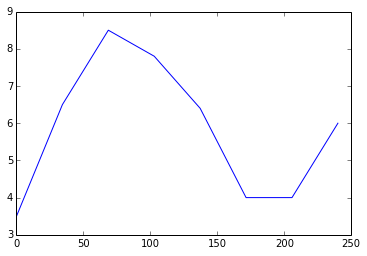## Example 4.23 pg : 166¶

In :
import math
from numpy import array,zeros_like

#Given
r = array([0, 8, 22, 74, 92, 105, 114, 120],dtype=float64);      				#raccumulated rainfall
T = array([0, 2, 4, 6, 8, 10, 12, 14],dtype=float64);            				#time for start of rainfall
V = 2e6;                             				#volume of run-off
A = 40.;                              				#catchment area
tr = 14.;                             				#duration of rainfall

# Calculations
d = V*1000/(40*1000000);

l = r-d;
W = l/tr;
I = zeros_like(r)
for i in range(1,8):
I[i] = r[i]-r[i-1];           				#incremental rainfall

#rainfall excess is available in 4 time intervals of 2 hrs
tre = 8.;
fi = (l-I-I-I)/tre;
fi = round(fi*100)/100;

# Results
print "fi index = %.2f mm/hr."%(fi);

fi index = 5.88 mm/hr.


## Example 4.24 pg : 167¶

In :
import math
from numpy import array

#Given
r = array([2.0 ,2.5, 7.6, 3.8, 10.6, 5.0, 7.0, 10.0, 6.4, 3.8, 1.4, 1.4]);     				#rainfall depths
R = 25.5;

# Calculations
s = sum(r)
tf = s-R;
af = tf/12;
#rainfall is less than average infiltration in1st,2nd,11th and 12th hours

f = (tf-r-r-r-r)/8;
f = round(f*10)/10;

# Results
print "average infiltration index = %d cm/hour."%(f);

average infiltration index = 3 cm/hour.


## Example 4.25 pg : 167¶

In :
import math
from numpy import zeros,zeros_like,array

#Given
r = array([2.0, 2.5, 7.6, 3.8, 10.6, 5.0, 7.0, 10.0, 6.4, 3.8, 1.4, 1.4]);     				#rainfall depths
A1 = 20.;
A2 = 40.;
A3 = 60.;

# Calculations and Results
A = A1+A2+A3;
fi1 = 7.6;
fi2 = 3.8;
fi3 = 1.0;
R1 = zeros_like(r)
R2 = zeros_like(r)
R3 = zeros_like(r)
for i in range(12):
R1[i] = r[i]-fi1;             				#rainfall excess
R2[i] = r[i]-fi2;
R3[i] = r[i]-fi3;
if (R1[i]<0):
R1[i] = 0;
if (R2[i]<0):
R2[i] = 0;
if (R3[i]<0):
R3[i] = 0;

print "average depth of hourly rainfall excesscm/hr";
a1 = zeros(12)
a2 = zeros(12)
a3 = zeros(12)
T = zeros(12)
for i in range(12):
a1[i] = R1[i]*A1/A;                    				#average rainfall excess
a2[i] = R2[i]*A2/A;
a3[i] = R3[i]*A3/A;
T[i] = a1[i]+a2[i]+a3[i];               				#total hourly rainfall excess
T[i] = round(T[i]*100)/100;
print "%.2f"%(T[i]);

average depth of hourly rainfall excesscm/hr
0.50
0.75
4.57
1.40
7.57
2.40
4.07
6.97
3.57
1.40
0.20
0.20


## Example 4.26 pg : 185¶

In :
from numpy import array,linspace
#derive the unit hydrograph

#Given
A = 92.;                   				#area of drainage bamath.sin
t = array([6, 8, 10, 12, 14, 16, 18, 20, 22, 24, 2, 4, 6, 8, 10, 12, 14, 16],dtype=float64);   				#time
r = array([10.6, 9.7, 107.8, 175.6, 193.9, 150.3, 126.2, 106.9, 90, 72.8, 58.2, 48, 36.2, 28.4, 20.2, 14, 10.2, 10.4]); 				#total run-off
B = array([10.6, 9.7, 9.73, 9.77, 9.8, 9.83, 9.87, 9.9, 9.93, 9.97, 10, 10.03, 10.07, 10.10, 10.13, 10.16, 10.20, 10.40]);  				#base flow
s = 0;

# Calculations and Results
d = r - B
s = sum(d)

n = 0.36*s*2/A;
print "ordinates of unit hydrograph:";
u = zeros(18)
for i in range(18):
u[i] = d[i]/n;                  				#ordinates of unit hydrograph
u[i] = round(u[i]*100)/100;
print "%.2f"%(u[i]);

print "Hydograph is 4-hr unit hydrograph";

ordinates of unit hydrograph:
0.00
0.00
11.50
19.45
21.60
16.48
13.65
11.38
9.39
7.37
5.65
4.45
3.07
2.15
1.18
0.45
0.00
0.00
Hydograph is 4-hr unit hydrograph


## Example 4.27 pg : 186¶

In :
import math
from numpy import zeros

#Given
A = 316.;         				#drainage area
B = 17.;          				#base flow
t = 6.;
O = [17.0, 113.2, 254.5, 198.0, 150.0, 113.2, 87.7, 67.9, 53.8, 42.5, 31.1, 22.6, 17.0];    				#ordinates of storm hydrograph
Or = zeros(13)
Oh = zeros(13)
for i in range(13):
Or[i] = O[i]-B;                 				#ordinates of direct run-off
Oh[i] = Or[i]/6.477;            				#ordinates of unit hydrograph

s = sum(Or);
re = s*60*60*t/(A*10000);
re = round(re*1000)/1000;

# Results
print "rainfall excess = %.2f cm."%(re);

rainfall excess = 6.48 cm.


## Example 4.28 pg : 188¶

In :
import math
from numpy import zeros

#Given
fi = 2.5;          				#infiltration index
B = 10;            				#Base flow
O = [0, 110, 365, 500, 390, 310, 250, 235, 175, 130, 95, 65, 40, 22, 10, 0, 0, 0];   				#ordinates of unit hydrograph
R1 = 2;R2 = 6.75;R3 = 3.75;
r1 = (R1*10-(fi*3)-5)/10;             				#rainfall excess in first three hour
r2 = (R2*10-(fi*3))/10;               				#rainfall excess in second three hour
r3 = (R3*10-(fi*3))/10;               				#rainfall excess in third three hour

s1 = zeros(18)
for i in range(18):
s1[i] = r1*O[i];
s2 = zeros(18)
for i in range(1,18):
s2[i] = r2*O[i-1];
s3 = zeros(18)
for i in range(2,18):
s3[i] = r3*O[i-2];
#surface run-off from rainfall excess during succesive unit periods
print "ordinates of storm hydrograph";
T = zeros(18)
t = zeros(18)
for i in range(18):
T[i] = s1[i]+s2[i]+s3[i];
t[i] = T[i]+B;
t[i] = round(t[i]*10)/10;
print "%.2f"%(t[i]);

ordinates of storm hydrograph
10.00
92.50
943.80
2905.00
4397.50
4082.50
3227.50
2616.30
2301.30
1862.50
1386.30
1018.80
715.00
461.50
269.50
136.00
40.00
10.00


## Example 4.29 pg : 189¶

In :
%matplotlib inline
from numpy import array,linspace,zeros
from matplotlib.pylab import plot,xlabel,ylabel
#derive and plot 6 hr unit hydrograph

#Given
A = 103.4;      				#area of bamath.sin
t = linspace(0,36,9);   				#time
q = [0, 21, 80, 82, 189, 123, 184, 87, 55.5, 25.25, 9, 6, 0];  				#flow
print "ordinates of unit hydrograph are:";
u = zeros(9)
u = 0;
u = q/2.;
u = (q-4*u)/2;
u = (q-4*u)/2;
for i in range(4,9):
u[i] = (q[i]-3*u[i-4]-4*u[i-2])/2;      				#ordinates of unit hydrograph

for i in range(9):
print "%.2f"%(u[i]);
print "The succesive unit hydrograph will have same ordinates but will be shiftedlaterally by 6 hrs.";
#graph is plotted between u and t.
plot(t,u)
xlabel("Time in hours")
ylabel("Discharge")

ordinates of unit hydrograph are:
0.00
10.50
40.00
20.00
14.50
5.75
3.00
2.00
0.00
The succesive unit hydrograph will have same ordinates but will be shiftedlaterally by 6 hrs.

Out:
<matplotlib.text.Text at 0x107903850>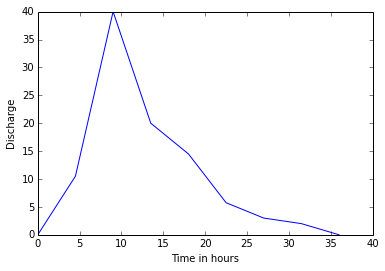## Example 4.30 pg : 190¶

In :
import math
from numpy import zeros

#derive ordinates of 6 hrs unit hydrograph

#Given
R = [0, 1, 2.7, 5, 8, 9.8, 9, 7.5, 6.3, 5, 4, 2.9, 2.1, 1.3 ,0.5, 0, 0, 0, 0, 0];  				#2hrs unit hydrograph
print "ordinates of 6 hrs unit hydrograph";
O1 = zeros(20)
for i in range(18):
O1[i+2] = R[i];
O2 = zeros(20)
for i in range(16):
O2[i+4] = R[i];
S = zeros(20)
f = zeros(20)#offset unit hydrograph
for i in range(20):
S[i] = O1[i]+O2[i]+R[i];        				#sum
f[i] = S[i]/3;                  				#ordinates of 6 hrs unit hydrograph
f[i] = round(f[i]*10)/10;
print "%.2f"%(f[i]);

ordinates of 6 hrs unit hydrograph
0.00
0.30
0.90
2.00
3.60
5.30
6.60
7.40
7.80
7.40
6.40
5.10
4.10
3.10
2.20
1.40
0.90
0.40
0.20
0.00


## Example 4.31 pg : 190¶

In :
import math
from numpy import array,linspace,zeros

#Given
t = linspace(0,45,16)     				#time
O = [0 ,9, 20, 35, 49, 43, 35, 28, 22, 17, 12, 9, 6, 3, 0, 0];           				#ordinate of 2 hr unit hydrograph

# Calculations and Results
of = zeros(16)
for i in range(2,16):
of[i] = O[i-2]+of[i-2];                 				#offset ordinate

s = zeros(16)
for i in range(16):
s[i] = O[i]+of[i];                       				#ordinate of s-curve

of1 = zeros(16)
for i in range(3,16):
of1[i] = s[i-3];                        				#offset of s-curve

print "ordinates of 9 hrs unit hydrograph:";
y = zeros(16)
u = zeros(16)
for i in range(16):
y[i] = s[i]-of1[i];
u[i] = 2*y[i]/3;                            				#ordinate of 9 hrs unit hydrograph
u[i] = round(u[i]*10)/10;
print "%.2f"%(u[i]);

ordinates of 9 hrs unit hydrograph:
0.00
6.00
13.30
29.30
40.00
44.70
40.00
30.70
26.00
18.70
15.30
10.00
8.00
4.00
2.00
0.00


## Example 4.32 pg : 210¶

In :
import math
from numpy import zeros,linspace

#california method
#Hazens method
#gumbels method

#Given
q = [9200, 7800, 6600, 5800, 5260, 4980, 4525, 3810, 3630, 3250, 3110, 3090, 2380, 2390, 1723]; 				#Discharge arranged in decreamath.sing order
N = 15;
C = 0.3;
m = linspace(1,15,15)
C = [0.3, 0.44, 0.52, 0.57, 0.61, 0.66, 0.7, 0.74, 0.78, 0.82, 0.86, 0.88, 0.94, 0.96, 1];   				#from table 4.25
print "California          Hazen          Gumbel";
Ca = zeros(15)
H = zeros(15)
G = zeros(15)
Ca = zeros(15)
G = zeros(15)

for i in range(15):
Ca[i] = N/m[i];
H[i] = 2*N/(2*m[i]-1);
G[i] = N/(m[i]+C[i]-1);
Ca[i] = round(Ca[i]*100)/100;
G[i] = round(G[i]*100)/100;
H[i] = round(H[i]*100)/100;
print "%.2f          %.2f          %.2f"%(Ca[i],H[i],G[i]);

California          Hazen          Gumbel
15.00          30.00          50.00
7.50          10.00          10.42
5.00          6.00          5.95
3.75          4.29          4.20
3.00          3.33          3.25
2.50          2.73          2.65
2.14          2.31          2.24
1.88          2.00          1.94
1.67          1.76          1.71
1.50          1.58          1.53
1.36          1.43          1.38
1.25          1.30          1.26
1.15          1.20          1.16
1.07          1.11          1.07
1.00          1.03          1.00


## Example 4.33 pg : 211¶

In :
import math

#Given
T1 = 40.;
T2 = 80.;            				#Return period
F1 = 27000.;
F2 = 31000.;      				#Peak flood

# Calculations
y80 = -(2.303*math.log10(2.303*math.log10(T2/(T2-1))));
y40 = -(2.303*math.log10(2.303*math.log10(T1/(T1-1))));
y = (F2-F1)/(y80-y40);
T = 240.;
y240 = -(2.303*math.log10(2.303*math.log10(T/(T-1))));
x240 = F2+(y240-y80)*y;

# Results
print "flood magnitude with return period of 240 years = %i cumec."%(x240);

flood magnitude with return period of 240 years = 37306 cumec.


## Example 4.34 pg : 212¶

In :
import math

#Given
N = 40;
Sn = 1.1413;
yn = 0.5436;         				#from table 4.21 (a) and(b)
q = [1330, 1095, 1030, 980, 975, 950, 945, 940, 925, 855, 853, 840, 835, 825, 810, 795, 756, 710, 708, 705, 700, 670, 625, 620, 610, 605, 595, 585, 570, 550, 530, 505, 500, 495, 485, 465, 460, 420, 390, 380];  				#discharge
s = sum(q)
xavg = s/N;
w = 0;

# Calculations
t = zeros(40)
for i in range(40):
t[i] = (q[i]-xavg)**2;
w = w+t[i];

sigma = (w/(N-1))**0.5;
N = 10.;
y10 = -(2.303*math.log10(2.303*math.log10(N/(N-1))));
K10 = (y10-yn)/Sn;
x10 = xavg+K10*sigma;
N = 20.;
y20 = -(2.303*math.log10(2.303*math.log10(N/(N-1))));
K20 = (y20-yn)/Sn;
x20 = xavg+K20*sigma;
N = 5.;
y5 = -(2.303*math.log10(2.303*math.log10(N/(N-1))));
K5 = (y5-yn)/Sn;
x5 = xavg+K5*sigma;

T = 100.;
y100 = -(2.303*math.log10(2.303*math.log10(T/(T-1))));
K100 = (y100-yn)/Sn;
x100 = xavg+K100*sigma;

T = 200.;
y200 = -(2.303*math.log10(2.303*math.log10(T/(T-1))));
K200 = (y200-yn)/Sn;
x200 = xavg+K200*sigma;
x100 = round(x100);

# Results
print "For T = 100 years:flood discharge = %.2f cumecs.\
\nFor T = 200 years:flood discharge = %.f cumecs."%(x100,x200);

For T = 100 years:flood discharge = 1487.00 cumecs.
For T = 200 years:flood discharge = 1620 cumecs.


## Example 4.35 pg : 214¶

In :
import math
from numpy.linalg import solve

#Given
sigma = 1.1413;        				#smath.radians(numpy.arcmath.tan(ard deviation
yn = 0.5436;
T = 50.;

# Calculations
y50 = -2.303*math.log10(2.303*math.log10(T/(T-1)));
K50 = (y50-yn)/sigma;
T = 100.;
y100 = -2.303*math.log10(2.303*math.log10(T/(T-1)));
K100 = (y100-yn)/sigma;
x50 = 878;  x100 = 970;
#Given peak flood
A = [[K50, 1],[K100, 1]];
B = [x50,x100];
C = solve(A,B)#A\B;
xavg = C;
sigmad = C;
T = 200.;
y200 = -2.303*math.log10(2.303*math.log10(T/(T-1)));
K200 = (y200-yn)/sigma;
x200 = xavg+K200*sigmad;
x200 = round(x200);

# Results
print "200 year flood for stream = %.2f cumecs."%(x200);

200 year flood for stream = 1062.00 cumecs.


## Example 4.36 pg : 214¶

In :
import math
#risk of failure of cofferdam
#return period

#Given
T = 30.;             				#deign for period
n = 6.;              				#period of construction

# Calculations
R = (1-(1-(1/T))**n)*100;
R1 = 0.1;          				#reduced risk
T1 = 1./(1-(1-R1)**(1./6));
R = round(R*10)/10;
T1 = round(T1*100)/100;

# Results
print "risk of failure of cofferdam = %.2f percent."%(R);
print "return period = %.2f years."%(T1);

risk of failure of cofferdam = 18.40 percent.
return period = 57.45 years.


## Example 4.37 pg : 215¶

In :
import math

#probability of excedence
#probability of flood magnitude occuring at:
#at least once in 10 years
#two times in 10 succesive years
#once in 10 succesive years

#Given
T = 40.;            				#return period
P = 1./T;
n = 10;
Rsk = 1.-(1-P)**n;
s = 1.;
t = 1.;
for i in range(1,n+1):
s = s*i;

for i in range(1,n-1):
t = t*i;

P2n = s*P**2*(1-P)**8/(t*2);
P1n = n*P*(1-P)**(n-1);
Rsk = round(Rsk*1000)/1000;
P2n = round(P2n*10000)/10000;
P1n = round(P1n*1000)/1000;

# Results
print "probability of excedence = %.2f."%(P);
print "probability of flood magnitude occuring at least once in 10 years = %.2f"%(Rsk);
print "probability of flood magnitude occuring at two times in 10 succesive years = %.2f"%(P2n);
print "probability of flood magnitude occuring at once in 10 succesive years = %.2f"%(P1n);

probability of excedence = 0.03.
probability of flood magnitude occuring at least once in 10 years = 0.22
probability of flood magnitude occuring at two times in 10 succesive years = 0.02
probability of flood magnitude occuring at once in 10 succesive years = 0.20


## Example 4.38 pg : 215¶

In :
import math

#Given
C1 = 0.22;C2 = 0.12;C3 = 0.32;     				#run-off coefficient
A1 = 3.2;A2 = 4.8;A3 = 1.8;
L = 2.4;                      				#length of water course
H = 30;                       				#fall
T = 30;                       				#frequency

# Calculations
t = 60*0.000323*(L*1000)**0.77*(H/(L*1000))**(-0.385);
i = 78*T**0.22/(t+12)**0.45;
q = 2.778*i*(C1*A1+C2*A2+C3*A3);
q = round(q*10)/10;

# Results
print "peak rate of run off = %.2f cumecs."%(q);

peak rate of run off = 141.20 cumecs.


## Example 4.39 pg : 216¶

In :
import math

#Given
T = 30;            				#return period
A = 2.4;           				#area of watershed
s = 1./200;         				#slope oof catchment
L = 1.8;           				#length of travel of water
C = 0.25;          				#average run-off coefficient
r = [2.5, 3.8, 4.8, 5.9, 6.7, 7.4, 8.4, 8.7, 9.2];   				#rmath.sinfall depth

# Calculations
t = 60*0.000323*(L*1000)**0.77*(s)**(-0.385);
rmax = r+(r-r)*7.84/10;
i = rmax*60/t;
q = 2.778*C*A*i;
q = round(q*100)/100;

# Results
print "peak flow rate = %.2f cumecs."%(q);

peak flow rate = 18.05 cumecs.


## Example 4.40 pg : 216¶

In :
import math

#Given
pA = 75.;         				#precipitation at A
pB = 58;         				#precpitation at B
pC = 47;         				#precipitation at C
nA = 826;        				#normal precipitation at A
nB = 618;        				#normal precipitation at B
nC = 482;        				#normal precipitation at C
nX = 757;        				#normal precipitation at X

# Calculations
pX = (nX*pA/nA+nX*pB/nB+nX*pC/nC)/3.;
pX = round(pX*10)/10;

# Results
print "precipitation at x = %.2f cm."%(pX);

precipitation at x = 70.90 cm.


## Example 4.41 pg : 216¶

In :
import math

#Given
p = [41, 51, 32, 55, 50, 68];   				#rain guage readings at respective stations
s = sum(p)
pavg = s/6;
u = 0;
for i in range(5):
u = u+(p[i]-pavg)**2;

# Calculations
sx = (u/5)**0.5;
Cv = sx*100/pavg;
N = (Cv/8)**2;
N = round(N*100)/100;

# Results
print "mean rainfall = %.2f cm."%(pavg);
print "total stations needed = %.2f."%(N);

mean rainfall = 49.00 cm.
total stations needed = 5.08.


## Example 4.42 pg : 217¶

In :
import math

#Given
a = 4;               				#dimension of plot sides
P1 = 4.8;P2 = 13;P3 = 8;P4 = 5.4;P5 = 3.2;P6 = 9.4;     				#precipitaion at respective stations

# Calculations
A1 = a**2/8+a**2/(4*1.73);
A2 = a**2/8;
A3 = A2;A4 = A1;
A5 = a**2/(4*1.73);
A6 = a**2/2;
A = A1+A2+A3+A4+A5+A6;
Pavg = (P1*A1+P2*A2+P3*A3+P4*A4+P5*A5+P6*A6)/A;

# Results
print "Mean precipitaion = %.2f cm."%(Pavg);

Mean precipitaion = 7.35 cm.


## Example 4.43 pg : 218¶

In :
import math
from numpy import linspace

#Given
A = [90, 140, 125, 140, 85, 40, 20];  				#area of isohytes
I = linspace(13,1,7)                  				#average isohytel interval
s = 0;t = 0;
for i in range(7):
s = s+A[i]*I[i];
t = t+A[i];

Pavg = s/t;
Pavg = round(Pavg*10)/10;

# Results
print " average depth of precipitation = %.2f cm."%(Pavg);

 average depth of precipitation = 8.40 cm.


## Example 4.44 pg : 218¶

In :
import math

#Given
p = [120, 95, 96, 60, 65, 70, 45, 21];   				#rain guage readings at respective stations

# Calculations and Results
s = sum(p)
pavg = s/8;
u = 0;
for i in range(8):
u = u+(p[i]-pavg)**2;

sx = (u/7)**0.5;
Cv = sx*100/pavg;
N = (Cv/13.99)**2;
N = round(N*100)/100;
print "mean rainfall = %.2f cm."%(pavg);
print "total stations needed = %.2f."%(N);
#taking N = 10
N = 10;
n = N-8;
print "additional guages needed = %i."%(n);

mean rainfall = 71.00 cm.
total stations needed = 10.03.
additional guages needed = 2.


## Example 4.45 pg : 219¶

In :
%matplotlib inline
from matplotlib.pylab import plot
from numpy import zeros,linspace
import math
#compute maximum rainfall intensities for 5,10,15,20,25,30,35,40,45,50 minutes
#plot intensity duration graph

#Given
CR = [0, 1.02, 2.08, 3.30, 4.72, 5.58, 6.40, 7.16, 7.88, 8.54, 9.14];  				#cumulative rainfall

c5 = zeros(11)
c10 = zeros(11)
c15 = zeros(11)
c20 = zeros(11)
c25 = zeros(11)
c30 = zeros(11)
c35 = zeros(11)
c40 = zeros(11)
c45 = zeros(11)
c50 = zeros(11)

c5 = CR;
c10 = CR;
c15 = CR;
c20 = CR;
c25 = CR;
c30 = CR;
c35 = CR;
c40 = CR;
c45 = CR;
c50 = CR;
for i in range(2,11):
c5[i] = CR[i]-CR[i-1];

for i in range(3,11):
c10[i] = CR[i]-CR[i-2];

for i in range(4,11):
c15[i] = CR[i]-CR[i-3];

for i in range(5,11):
c20[i] = CR[i]-CR[i-4];

for i in range(6,11):
c25[i] = CR[i]-CR[i-5];

for i in range(7,11):
c30[i] = CR[i]-CR[i-6];

for i in range(8,11):
c35[i] = CR[i]-CR[i-7];

for i in range(9,11):
c40[i] = CR[i]-CR[i-8];

for i in range(10,11):
c45[i] = CR[i]-CR[i-9];
#rainfall in any possible time interval

print "5min    10min    15min    20min    25min    30min    35min    40min    45min    50min";
for i in range(11):
print "%4.2f    %5.2f    %5.2f    %5.2f    %5.2f    %5.2f    %5.2f    %5.2f    %5.2f    %5.2f"%(c5[i],c10[i],c15[i],c20[i],c25[i],c30[i],c35[i],c40[i],c45[i],c50[i]);

I = [17.04, 15.84, 14.80, 14.16, 13.39, 12.80, 12.27, 11.82, 11.39, 10.97];    				#maximum intensity at respective durations
D = linspace(5,50,len(I))               				#durations
#graph is plotted between I and D
plot(I,D)

5min    10min    15min    20min    25min    30min    35min    40min    45min    50min
0.00     0.00     0.00     0.00     0.00     0.00     0.00     0.00     0.00     0.00
1.02     0.00     0.00     0.00     0.00     0.00     0.00     0.00     0.00     0.00
1.06     2.08     0.00     0.00     0.00     0.00     0.00     0.00     0.00     0.00
1.22     2.28     3.30     0.00     0.00     0.00     0.00     0.00     0.00     0.00
1.42     2.64     3.70     4.72     0.00     0.00     0.00     0.00     0.00     0.00
0.86     2.28     3.50     4.56     5.58     0.00     0.00     0.00     0.00     0.00
0.82     1.68     3.10     4.32     5.38     6.40     0.00     0.00     0.00     0.00
0.76     1.58     2.44     3.86     5.08     6.14     7.16     0.00     0.00     0.00
0.72     1.48     2.30     3.16     4.58     5.80     6.86     7.88     0.00     0.00
0.66     1.38     2.14     2.96     3.82     5.24     6.46     7.52     8.54     0.00
0.60     1.26     1.98     2.74     3.56     4.42     5.84     7.06     8.12     9.14

Out:
[<matplotlib.lines.Line2D at 0x107a3ca50>]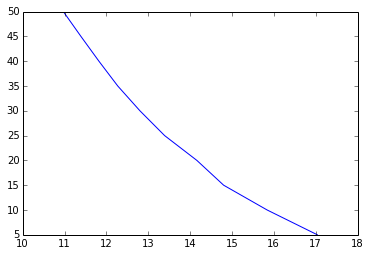## Example 4.46 pg : 221¶

In :
%matplotlib inline
import math
from matplotlib.pylab import plot
#draw storm hyetograph and intensity duration curve

#Given
p = [0, 5, 7.5, 8.5, 9];      				#accumulated precipitation
t = [0, 30, 60, 90, 120];     				#time
r = zeros(5)
I = zeros(5)

print "Rainfall intensity:";
for i in range(1,5):
r[i] = p[i]-p[i-1];          				#rainfall in succesive 30 min interval
I[i] = r[i]*60/30;           				#rainfall intensity
print "%.2f"%(I[i]);

#graph is plotted between I and t.
plot(I,t)

Rainfall intensity:
10.00
5.00
2.00
1.00

Out:
[<matplotlib.lines.Line2D at 0x107a6a850>]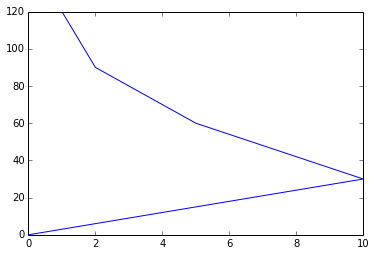## Example 4.47 pg : 222¶

In :
%matplotlib inline
import math
from numpy import zeros,linspace

#Given
I = linspace(21,12,10)          				#isohytes
a = [543, 1345, 2030, 2545, 2955, 3280, 3535, 3710, 3880, 3915];  				#enclosed area
ia = zeros(10)
ia = 543;
for i in range(1,10):
ia[i] = a[i]-a[i-1];              				#net incremental area between isohytes

rv = zeros(10)
r = linspace(21.5,12.5,10)
for i in range(10):
rv[i] = r[i]*ia[i];               				#rainfall volume

cv = zeros(10)
cv = 11675;
for i in range(10):
cv[i] = cv[i-1]+rv[i];            				#cumulative volume

eud = zeros(10)
for i in range(10):
eud[i] = cv[i]/a[i];               				#depth(mm)

print "From depth area curve we obtain average depth of precipitation = 20.15 mm for anarea of 2400 sq. km.";
#graph is plotted between eud and a
plot(eud,a)

From depth area curve we obtain average depth of precipitation = 20.15 mm for anarea of 2400 sq. km.

Out:
[<matplotlib.lines.Line2D at 0x107afa410>]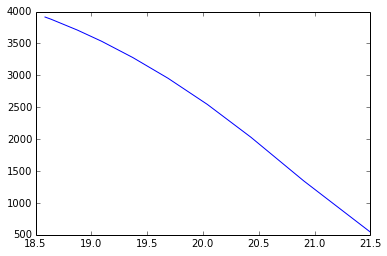## Example 4.48 pg : 222¶

In :
import math

#Given
h1 = 7.75;        				#initial depth of water
r = 3.80;         				#rainfall during the week
hr = 2.50;       				#depth of water removed
C = 0.7;        				#pan coefficient

# Calculations
ha = r-hr;
hl = ha+h1;
h2 = 8.32;
ev = hl-h2;
evs = ev*C;
evs = round(evs*100)/100;

# Results
print "evaporation from reservior surface during the week = %.2f cm."%(evs);

evaporation from reservior surface during the week = 0.51 cm.


## Example 4.49 pg : 223¶

In :
import math
from numpy import linspace

#Given
T = linspace(1,12,12)      				#time from start
r = [1.8, 2.6, 7.8, 3.9, 10.6, 5.4, 7.8, 9.2, 6.5, 4.4, 1.8, 1.6];  				#increamental rainfall
R = 24.4;        				#total run-off
s = sum(r)

ti = s-R;

#first trial
tr = 7;   				#assumed
ti = s-R-r-r-r-r-r;
fi = ti/tr;
P = zeros(12)
for i in range(12):
P[i] = r[i]-fi;
if (P[i]<0):
P[i] = 0;

print "Timeh          rainfall excess.";
for i in range(12):
print "%.2f          %.2f"%(T[i],P[i]);

print "fi index = %.2f cm/hr."%(fi);

Timeh          rainfall excess.
1.00          0.00
2.00          0.00
3.00          3.90
4.00          0.00
5.00          6.70
6.00          1.50
7.00          3.90
8.00          5.30
9.00          2.60
10.00          0.50
11.00          0.00
12.00          0.00
fi index = 3.90 cm/hr.


## Example 4.50 pg : 224¶

In :
import math
from numpy import linspace

#Given
r = [0.6, 1.35, 2.25, 3.45, 2.7, 2.4, 1.5, 0.75];      				#incremental rainfall
T = linspace(1,8,8)            				#time from start of rainfal
t = 8.;
P = 15.;                				#total rainfall
R = 8.7;              				#direct run-off

# Calculations
W = (P-R)/t;
#math.since fi wil be more than W
tre = 6;
fi = ((P-R)-r-r)/tre;

# Results
print "fi index = %.2f cm/hr."%(fi);

fi index = 0.83 cm/hr.


## Example 4.51 pg : 224¶

In :
import math
from scipy.integrate import quad

#Given
I = 10;           				#total infiltration rate
fI = 5;           				#final infiltration rate
k = 0.95;         				#rate of decay of difference between final and initial infiltration rate

# Calculations
def f8(t):
return fI+(I-fI)*math.e**(-k*t)

q =  quad(f8,0,6)
q = round(q*100)/100;

# Results
print "total infiltration depth = %.2f mm."%(q);

total infiltration depth = 35.25 mm.


## Example 4.52 pg : 224¶

In :
import math
from numpy import linspace

#find the equation of infiltration capacity

#Given
fc = 1;            				#consmath.tant infiltration rate
ft = [10.4, 5.6, 3.2, 2.1, 1.5, 1.2, 1.1, 1, 1];   				#infiltration capacity
f = ft-fc;
t = linspace(0,2,9)

r = zeros(9)
for i in range(9):
r[i] = ft[i]-fc;

h = zeros(7)
for i in range(7):
h[i] = math.log10(r[i]);

s = 0.775;        				#from graph
k = 1/(math.log10(math.e)*s);
k = round(k*100)/100;

# Results
print "Equation is:ft = fc+%.2fe**-%.2ft)"%(f,k);

Equation is:ft = fc+9.40e**-2.97t)


## Example 4.53 pg : 226¶

In :
%matplotlib inline
from matplotlib.pylab import bar
import math
#total rainfall
#net run-off
#W index

#Given
r = [2, 2, 8, 7, 1.25, 1.25, 4.5];       				#rainfall intensity
T = [15, 30, 45, 60, 70, 90, 105];                        				#time
dt = 15.;                              				#time interval
fi = 3.;                               				#fi index
#graph is plotted between r and T
bar(T,r)
s = sum(r)
P = s*dt/60;
Pe = ((8-3)+(7-3)+(4.5-3))*dt/60;    				#area of graph above r = 3.0.
w = (P-Pe)/(105./60);
w = round(w*1000)/1000;

# Results
print "total rainfall = %.2f cm."%(P);
print "net run-off = %.2f cm."%(Pe);
print "W index = %.2f cm/hr."%(w);

total rainfall = 6.50 cm.
net run-off = 2.62 cm.
W index = 2.21 cm/hr.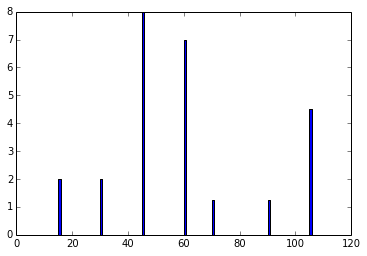## Example 4.54 pg : 226¶

In :
import math

#run-off by rainfall of 3.3cm in 3hrs

#Given
A = [36, 18, 66];             				#area of catchment
fi = [0.9 ,1.1, 0.5];         				#fi index
r1 = [0.6 ,0.9, 1.0];         				#rainfall in first hour
r2 = [2.4 ,2.1, 2.0];        				#rainfall in second hour
r3 = [1.3, 1.5, 0.9];        				#rainfall in third hour

# Calculations and Results
t36 = r1+r2+r3;
t18 = r1+r2+r3;
t66 = r1+r2+r3;

p = (t36*A+t18*A+t66*A)/(A+A+A);
print "Total rainfall in catchment = %.2f cm."%(p);

ro1 = [0 ,0, 0.5];
ro2 = [1.5 ,1.0, 1.5];
ro3 = [0.4, 0.4, 0.4];    				#rainfall-fi
t1 = ro1+ro2+ro3;
t2 = ro1+ro2+ro3;
t3 = ro1+ro2+ro3;
run = (A*t1+A*t2+A*t3)/(A+A+A);       				#run-off from entire catchment

print "run-off by rainfall of 3.3cm in 3hrs = %.2f cm."%(run);

fia = (fi*A+fi*A+fi*A)/(A+A+A);
tr = (1.1-fia)*3;
print "Total run-off = %.2f cm."%(tr);

Total rainfall in catchment = 4.11 cm.
run-off by rainfall of 3.3cm in 3hrs = 2.10 cm.
Total run-off = 1.17 cm.


## Example 4.55 pg : 227¶

In :
import math
from numpy import array

#Given
P = array([4, 22, 28, 15, 12, 8, 4, 15, 10, 5]);  				#Precipitation
R = array([0.2, 7.1, 10.9, 4.0, 3.0, 1.3, 0.4, 4.1, 2.0, 0.3]);  				#runoff

# Calculations
Ps = P**2
Rs = R**2
PR = P*R

s = sum(Ps)
t = sum(Rs)
u = sum(PR)
q = sum(P)
w = sum(R)

N = 10.;
a = (N*u-q*w)/(N*s-q**2);
b = (w-a*q)/N;
a = round(a*10000)/10000;
b = round(b*10000)/10000;

# Results
print "Equation is:%.2f P %.2f."%(a,b);

Equation is:0.43 P -1.93.


## Example 4.56 pg : 228¶

In :
import math

#Given
Q = 470;                				#peak discharge of flood hydrograph
B = 15;                 				#base flow
l = 0.25;               				#infiltration loss
Qr = Q-B;
d = 8;                  				#average depth of rainfall

# Calculations
re = d-l*6;            				#rainfall excess
q = Qr/re;

# Results
print "peak discharge of 6 hrs unit hydrograph = %i cumecs."%(q);

peak discharge of 6 hrs unit hydrograph = 70 cumecs.


## Example 4.57 pg : 228¶

In :
import math
from numpy import array,zeros

#Given
fi = 0.25;          				#infiltration index
B = 20;            				#Base flow
O = array([0, 20, 60, 150, 120, 90, 70, 50, 30, 20, 10, 0, 0, 0]);   				#ordinates of unit hydrograph
R1 = 5;
R2 = 0.8;
R3 = 3;
r1 = R1-(fi*4);                                 				#rainfall excess in first four hour
r2 = R2-(fi*4);                                 				#rainfall excess in second four hour
r3 = R3-(fi*4);                                 				#rainfall excess in third four hour
if r2<0 :
r2 = 0;

# Calculations and Results
s1 = r1*O
s2 = zeros(14)
for i in range(1,14):
s2[i] = r2*O[i-1];

s3 = zeros(14)
for i in range(2,14):
s3[i] = r3*O[i-2];
#surface run-off from rainfall excess during succesive unit periods
print "ordinates of storm hydrograph";
T = zeros(14)
t = zeros(14)
for i in range(14):
T[i] = s1[i]+s2[i]+s3[i];             				#sub-total
t[i] = T[i]+B;                        				#ordinate of flood hydrograph
print "%i"%(t[i]);

ordinates of storm hydrograph
20
100
260
660
620
680
540
400
280
200
120
60
40
20


## Example 4.58 pg : 229¶

In :
import math
from numpy import array

#Given
fi = 2.5;            				#fi index
t = 24.;
A = 200.;             				#area of catchment
R1 = 7.5;
R2 = 2.0;
R3 = 5.; 				#rainfall
r1 = R1-fi;
r2 = R2-fi;
r3 = R3-fi;
r2 = 0;
r = [5, 0, 2.5];      				#excess rainfall
D = array([5 ,15, 40, 25, 10, 5, 0, 0, 0]);  				#distribution
d1 = D*r/100
d2 = zeros(9)
for i in range(8):
d2[i+1] = D[i]*r/100;

d3 = zeros(9)
for i in range(7):
d3[i+2] = D[i]*r/100;
#distribution run-off for rainfall excess

tr1 = zeros(9)
tr2 = zeros(9)
for i in range(9):
tr1[i] = d1[i]+d2[i]+d3[i];           				#total run-off as depth
tr2[i] = 23.148*tr1[i];               				#total run-off as discharge
tr2[i] = round(tr2[i]*1000)/1000;

s = sum(tr2)

print "Total run-off:";
print "as depth          as discharge";
for i in range(9):
print "%.2f          %.2f"%(tr1[i],tr2[i]);

r = 0.36*s*t/A;
r = round(r*10)/10;
print "total run-off = %.2f cm."%(r);

Total run-off:
as depth          as discharge
0.00          0.00
0.00          0.00
2.12          49.19
1.38          31.83
1.00          23.15
0.62          14.47
0.25          5.79
0.12          2.89
0.00          0.00
total run-off = 5.50 cm.


## Example 4.59 pg : 230¶

In :
import math
from numpy import zeros

#Given
O = [10, 30, 90, 220, 280, 220, 166, 126, 92, 62, 40, 20, 10];      				#ordinates of 6 hr flood hydrograph
B = 10;          				#Base flow
r = zeros(13)

for i in range(13):
r[i] = O[i]-B;           				#ordinates of direct run-off

print "Ordinates of 6 hr unit hydrograph";
u = zeros(13)

for i in range(1,13):
u[i] = r[i]-u[i-1];          				#ordinates of 6 hrs unit hydrograph

for i in range(13):
print "%i"%(u[i]);

Ordinates of 6 hr unit hydrograph
0
20
60
150
120
90
66
50
32
20
10
0
0


## Example 4.60 pg : 231¶

In :
import math
from numpy import zeros

#determine the ordinates of 1 cm-6 hour hydrograph

#Given
t = 6;
A = 450;       				#catchment area
O = [5, 15, 40, 80, 60, 50, 25, 15, 5];   				#ordinates of flood hydrograph
B = 5;         				#base flow assumed
s = 0;
r = zeros(9)
for i in range(9):
r[i] = O[i]-B;        				#ordinates of direct run-off
s = s+r[i];

n = s*0.36*12/A;
print "ordinates of unit hydrograph";
for i in range(9):
u[i] = r[i]/n;
u[i] = round(u[i]*100)/100;
print "%.2f"%(u[i]);

ordinates of unit hydrograph
0.00
4.17
14.58
31.25
22.92
18.75
8.33
4.17
0.00


## Example 4.61 pg : 232¶

In :
import math
from numpy import zeros

#obtain ordinates 24 hr unit hydrograph

#Given
O = [0, 5.5, 13.5, 26.5, 45, 82, 162, 240, 231, 165, 112, 79, 57, 42, 31, 22, 14, 9.5, 6.6, 4, 2, 1, 0, 0, 0, 0, 0];  				#ordinates of  1st 8 hrs unit hydrograph
o1 = zeros(27)
o2 = zeros(29)
for i in range(25):
o1[i+2] = O[i];                          				#ordinates of  2nd 8 hrs unit hydrograph
o2[i+4] = O[i];                          				#ordinates of  3rd 8 hrs unit hydrograph

o3 = zeros(27)
t = zeros(27)
print "ordinates 24 hr unit hydrograph:";
for i in range(27):
o3[i] = o1[i]+o2[i]+O[i];        				#total 24 hr hydrograph of 3 cm run-off
t[i] = o3[i]/3;
t[i] = round(t[i]*10)/10;
print "%.2f"%(t[i]);

ordinates 24 hr unit hydrograph:
0.00
1.80
4.50
10.70
19.50
38.00
73.50
116.20
146.00
162.30
168.30
161.30
133.30
95.30
66.70
47.70
34.00
24.50
17.20
11.80
7.50
4.80
2.90
1.70
0.70
0.30
0.00


## Example 4.62 pg : 233¶

In :
%matplotlib inline
import math
from numpy import zeros,linspace

#ordinates of 1 hr unit hydrograph

#Given
t = linspace(0,12,13) 			#time
O = [0, 0, 54, 0, 175, 0, 127, 0, 58, 0, 25, 0, 0, 0];           				#ordinate of 2 hr unit hydrograph
of = zeros(13)
for i in range(2,13):
if (i%2) == 0:
of[i] = 0
else:
of[i] = O[i-2]+of[i-2];

s = [0, 25, 54, 120, 229, 300, 356, 390, 414, 430, 439, 439, 439];     				#Ordinates of S-curve
of1 = zeros(13)
for i in range(1,13):
of1[i] = s[i-1];

y = zeros(13)
u = zeros(13)
print "ordinates of 1 hr unit hydrograph:";
for i in range(13):
y[i] = s[i]-of1[i];
u[i] = y[i]*2;
print "%i"%(u[i]);

#graph is plotted between u and t
plot(t,u)

# graph in book is wrong. Please check.

ordinates of 1 hr unit hydrograph:
0
50
58
132
218
142
112
68
48
32
18
0
0

Out:
[<matplotlib.lines.Line2D at 0x107bea8d0>]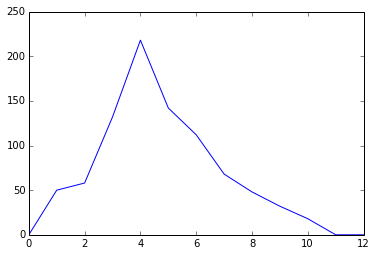## Example 4.63 pg : 234¶

In :
import math

#Given
xavg = 1200;        				#sample mean
n = 50.;             				#assurance year
A = 0.95;           				#assurance percent
Rsk = 1-A;
sigma = 650;        				#smath.radians(numpy.arcmath.tan(ard deviation
yn = 0.53622;       				#mean of reduced variate
sigma30 = 1.11238;  				#smath.radians(numpy.arcmath.tan(ard deviation of reduced variate

# Calculations
T = 1/(1-(1-Rsk)**(1/n));
yt = -2.303*math.log10(2.303*math.log10(T/(T-1)));
K = (yt-yn)/sigma30;
xt = xavg+K*sigma;

# Results
print " design disharge = %i cumecs."%(xt);

 design disharge = 4908 cumecs.


## Example 4.64 pg : 235¶

In :
import math

#Given
T1 = 50.;
T2 = 100.;            				#Return period
F1 = 20600.;
F2 = 22150;      				#Peak flood

# Calculations
y100 = -(2.303*math.log10(2.303*math.log10(T2/(T2-1))));
y50 = -(2.303*math.log10(2.303*math.log10(T1/(T1-1))));
y = (F2-F1)/(y100-y50);
T = 500.;
y500 = -(2.303*math.log10(2.303*math.log10(T/(T-1))));
x500 = F2+(y500-y100)*y;
x500 = round(x500);

# Results
print "flood magnitude with return period of 240 years = %.2f cumec."%(x500);

flood magnitude with return period of 240 years = 25732.00 cumec.


## Example 4.65 pg : 235¶

In :
import math

#Given
xavg = 1.65;      				#mean of data
sigma = 0.45;     				#smath.radians(numpy.arcmath.tan(ard deviation
x = 3;

# Calculations
y = 1.2825*(x-xavg)/sigma+0.577;
l = math.e**(math.e**(-y));
T = l/(l-1);
T = round(T*10)/10;

# Results
print "recurrence interval of 10 minutes storm = %.2f years."%(T);

recurrence interval of 10 minutes storm = 84.00 years.


## Example 4.66 pg : 236¶

In :
import math

#Given
T1 = 50.;
T2 = 100.;            				#Return period
F1 = 30800.;
F2 = 36300.;      				#Peak flood

# Calculations
y100 = -(2.303*math.log10(2.303*math.log10(T2/(T2-1))));
y50 = -(2.303*math.log10(2.303*math.log10(T1/(T1-1))));
y = (F2-F1)/(y100-y50);
T = 200.;
y200 = -(2.303*math.log10(2.303*math.log10(T/(T-1))));
x200 = F2+(y200-y100)*y;
x200 = round(x200);

# Results
print "flood magnitude with return period of 240 years = %.2f cumecs."%(x200);

flood magnitude with return period of 240 years = 41780.00 cumecs.


## Example 4.67 pg : 236¶

In :
import math

#Given
xavg = 4200.;      				#mean
sigma = 1705.;     				#smath.radians(numpy.arcmath.tan(ard deviation
xt = 9550.;        				#flood value

# Calculations
K = (xt-xavg)/sigma;
yt = 1.2825*K+0.577;
l = math.e**(math.e**(-yt));
T = l/(l-1);

# Results
print "Return period of flood of 9950 cumec/s = %.2f years."%(T);

Return period of flood of 9950 cumec/s = 100.11 years.


## Example 4.68 pg : 237¶

In :
import math

#Given
T1 = 100.;
T2 = 50.;            				#Return period
F1 = 485.;
F2 = 445.;      				#Peak flood

# Calculations
y50 = -(2.303*math.log10(2.303*math.log10(T2/(T2-1))));
y100 = -(2.303*math.log10(2.303*math.log10(T1/(T1-1))));
y = (F2-F1)/(y50-y100);
T = 1000.;
y1000 = -(2.303*math.log10(2.303*math.log10(T/(T-1))));
x1000 = F2+(y1000-y50)*y;
x1000 = round(x1000*10)/10;

# Results
print "flood magnitude with return period of 240 years = %.2f cumecs."%(x1000);

flood magnitude with return period of 240 years = 617.20 cumecs.


## Example 4.69 pg : 238¶

In :
import math

#probability of exceedence
#probability of occurence in next 12 years

#Given
T = 25.;        				#return period
n = 12.;

# Calculations
P = 1/T;
Rsk = 1-(1-P)**n;
P = round(P*100)/100;
Rsk = round(Rsk*10000)/10000;

# Results
print "probability of exceedence = %.2f."%(P);
print "probability of occurence in next 12 years = %.2f."%(Rsk);

probability of exceedence = 0.04.
probability of occurence in next 12 years = 0.39.